## Wednesday, January 27, 2010

### Jerick73 Scribepost for Percent Foldable

Yesterday, we had are homework was to copy down the key ideas in our foldable and show the recipes to find 10%, 5%, 1%, 25%, 150, 0.5%.

Key Ideas
You can use mental math strategies such as halving, doubling, and dividing by ten to find the percents of some numbers.

To calculate the percent of a number, write the percent as a decimal and then multiply by the number.

12.5 % of 50 =0.125 x 50 =6.25

Communicate the Ideas
1. Explain to a classmate how you could use mental math to find each of the following.
a)300% of 40 b)0.5 of 120 c)10.5% of 80

a)300% of 400 , 100% is 40 so you simply multiply 100% by 3 and you get 300%

b)0.5% of 120, Divide 120 by 100 to get 1% and divide by 2 to get half of 1 % which is 0.5%

c)10.5 of 80, Divide 80 by 10 to get the 10 percent , now you have your 10 , to get the .5 divide the 10% by 10 to get 1 % and half that one to get your .5% then add the numbers 10+.5

2. Describe two ways to find 6% of 120

- Divide by 10 to get 10% then divide by two to get 5% , after divide your 10% by 10 to get 1% and add 1%+5% to get your 6% number.

-Divide by 100 to get 1% and multiply that by 6 to get 6%

How To Find...
10% - Divide the Number by 10
5% - Divide by 10 then divide by 2
1% - Divide by 100
25% - Divide by 4
150% - Divide by 2 and add your 100% number with your 50% number
0.5% - Divide by 100 then Divide by 2

## Tuesday, January 26, 2010

### Eric.P's Percent Scribepost for January 26, 2010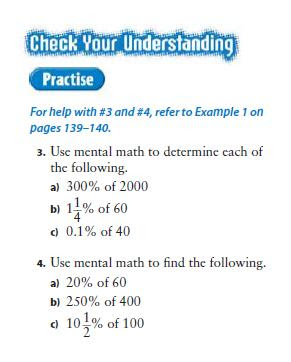Okay today's homework was to answer the questions 3- 6 to from page 142 in the math text book. Also you can't use a calculator everything has to be done in your head. If you use a calculator Harbeck will kick you in the leg.

So here's how I got question 3 and 4 using my head.
3.

A) To find 300% of 2000 you need to times 2000 by 3 because a percent is always out of 100 and 300% is 3 times larger than 100.

2000 x 3 = 6000
100% x 3 = 300%
6000 = 300%

B) To find 1 and one quarter of a percent of 60 you need to first find 1%. Now that you have 1 percent you can find 1 quarter of a percent by dividing it by 4. Now add both the 1 percent and the one quarter of the percent together to get your answer.

60 / 100 = 0.6
0.6 / 4 = 0.15
0.15 + 0.6 = 0.75
0.75 = 1 and one quarter of a percent.

Tip: For those who had trouble with figuring out 0.6 divided by 4 you can times it by 100 then divide by 4 then divide by 100 to get your answer.

c) To find 0.1% of 40 you need to divide 40 by 1000.
40 / 1000 = 0.04
Tip: Dividing by a number like this that has 1 then a bunch of zeros you can just move the decimal place left by how many zeros there are.

4.
A) To find 20% of 60 you need to divide 60 by 5. I will explain why you do this since 20% is 5 times smaller than 100% you will need to divide 60 by 5.
60 / 5 = 12

Tip: Another way to do this is to divide by 10 then times 2. You can do this because 100% divided by 10 is 10% then times 2 is 20%.

B) To find 250% of 400 you need to first times 400 by 2 to get 200%. Still using your 400 divide it by 2to get 50% add the 2 and you will get your answer.

400 x 2 = 800
400 / 2 = 200
800 + 200 = 1000

c) To find 10 and a half of a percent of 100 you need to first find 10% of 100. To find 10% just divide by 10. Now you need to find half a percent first with the 10% you have divide that by 10 again to get 1% now with that divide the 1% in half to get the half of a percent. Now add the 10% and the half of a percent together to get your answer.

100/ 10 = 10
10 / 10 = 1
1 / 2 = 0.5
10 + 0.5 = 10.5
If I messed up or something comment and tell me.

### Percent Scribe for January 26, 20105. a) 2/5% of 325 = 1.3
2/5% = 0.4%
First find 1% of 325. Do that by dividing 325 to 100 and you will get 3.25, then divide it again by 10 to get 0.1% (0.325) multiply 0.325 by 4. The answer is 1.3

b) 15 1/4% of 950 = 144.88
Find 10% of 950, 950÷ 10 = 95 (10%), divided it into half so 95 ÷ 2 = 47.5 (5%), 95 + 47.5 = 142.5.
950 ÷ 100 = 9.5 (1%) ÷ 4 = 2.375 (1/4%)
142.5 + 2.375 = 144.88

c) 175% of \$125.50 = 219.63
100% is 125.50 ÷ 2 to get 50%.
125.50 ÷ 2 = 62.75 (50%) divide it into 2to get 25%
62.75 ÷ 2 = 31.375(25%)
125.50 + 62.75 + 31.375 = 219.63

6. a) 5/8 of 520 = 3.25
5/8 = (0.625)
520 ÷ 100 = 5.2 (1%) ÷ 2 = 2.6 (0.5%) ÷ 2 = 1.3 (0.25%) ÷ 2 = 0.65(0.125)
2.6 + 0.65 = 3.25

b) 75 2/5% of 200 = 150.8
divide 200 by 4 to get 25%! 200 ÷ 4 = 50(multiply 50 by 3) 50 x 3 = 150 (75%)
divide 200 by 100 to get 1%! 200 ÷ 100 = 2(1%) (divide by 10) 2 ÷10 = 0.2 (0.1) multiply by 4 to get 2/5%. 0.2 x 4 = 0.8
150 + 0.8 = 150.8

c) 250% of \$76.50 = 191.25
Multiply 76.5 by 2. 76.5 x 2 = 153(200%)
divide 76.5 by 2. 76.5 ÷2 = 38.25

## Monday, January 25, 2010

### Vicky's Finding Percent Post

HEEEYYOO.

Today in class we were practicing how to find percent out of different numbers. Our homework was to find a variety of percents out of 400.

I did this all without a calculator. I did this all in my head. So thats why I dont have any of the work to show. Because some of the answers were kind of hard to write out and explain how I've done it.

I don't know if my answers are all that accurate, but I've double checked them on my calculator to see if they were correct. If you guys find any errors you can just comment .

Sorry if it's blurry and bright on one side and dark on the other. I had to use my webcam because my camera didn't work.

Hope you guys liked my post :) COMMENT PLEEEEASE .

## Tuesday, January 19, 2010

### Problems & Percents

Problems & Percents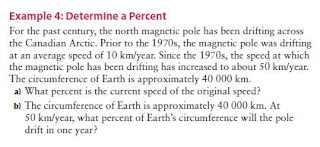We will use example 4: Determine a Percent
This example uses PROPORTIONS in this problem.
To solve this problem we will do part a.
a)What percent is the current speed of the original speed?
For this we will use proportions to help us.
We need to find the CURRENT speed over the ORIGINAL speed.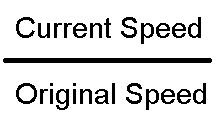Now we need to find the original speed and current speed.
The current speed is at 50 km/year.
The original speed was at 10 km/year.Now you can see here the current speed is 50 km over 10 km (Current Speed over Original Speed)
Now we need to find what percent of the current speed is over the original speed.
10 km is 100%
How do you get from 10 km to 50 km?
10 x 5 = 50 km
So we do the same thing we do to the percent.
100% x 5 = 500%Here is the answer to a.
The current speed is 500% over the
original speed.

Now we will do b.
In this question we're finding how much the pole drifts in 1 year around the earths circumference in percent form.
We know that the pole drifts 50 km / year.
The Earths circumference is 40 000 km.
We will also be using proportion for this.
We need to find the poles km over the earths circumference.
So it will be 50 km over 40 000 km.Now we will need to simplify this into lowest terms.
The answer we get is 1 / 800
Now we can find the percent by dividing 1 by 800.
1/800 = 0.00125
To get the percent we need to multiply the decimal by 100.
0.00125 x 100 = 0.125%.

So the answer is that at 50km / year the pole drifts 0.125% or 1/8% of the Earths circumference in 1 year.

## Monday, January 18, 2010

### Percents 2

As of January 17th, 10 we continue with our new unit "PERCENTS,FRACTIONS, AND DECIMALS". So for todays class we had to work on our foldable under (fractions, decimals, and percents). If you hadn't finished it yet .. that's what my scribe is here for to help you :) .

KEY IDEAS :

• Fractions, Decimals, and Percents can be used to represent numbers in various situations.
• Percents can be written as a fraction and as decimals.

1/2% = 0.5 150% = 150/100 42 3/4% = 42.75%

o.5% = 0.5/100 = 1.5 or 1 1/2 42.75 % = 42.75/100

= 0.005 = 0.4275

## Thursday, January 14, 2010

### Krystal`s Scribe for January. 14, 2010Decimal to Percent=You just have to move the decimal 2 places to the right.Another way to do it is to multiply it by 100. For example. 1.25X100 =125. Just add the percent sign. I find it way easier by doing it with multiplying by 100.

Fraction to Percent= The way I learned was a little different.Since fractions is the same as division, I just divide the fraction number and get the percentage. For example . 61 over 100= 61 divided by 100 = 0.61,just add the percent sign.

Decimal to Fraction= When a decimal has 2 numbers after the decimal it`s out of 100, if it only has 1 number after the decimal, its out of 10, if it has 3 numbers after the decimal it`s out of 1000.ect.For example. 0.005= 5 over 1000. But if its a decimal like this "3.2", 3 is the whole and 2 is out of 10 since it`s the only number after the decimal.the fraction would be , 3 as the whole and 2 over 10.Don`t forget to simplify so it would be , 3 as a whole and 1 over 5. To simplify it , just divide it by 2.

Fraction to Decimal= Remember, a fraction is division turned up-right. That means if the fraction was, 7 over 10, side-to-side would be, 7 divided by 10 =0.7 . Easy,right ? But what if your fraction has a whole number ? for example : 1 and 1 over 4, since the 1 over 4 is simplified, think of it as not simplified "25 over 100" = 25 divided by 100 = 0.25. Take the ".25" and do nothing to the whole number,Then you have "1.25". Sorry, I tried explaining it as best as I could. It`s really easy if you understand what I`m talking about.

BY THE WAY ! Sorry it`s late...

### Hannah's scribepost for January 13.

Sorry it's late.

question 4.question8.
How many hundred grids are needed to show each of the following percents?
a.) 300% = 3 hundreds grids

b.) 466% = 5 hundreds grids
c.) 1200% = 12 hundreds grids
question9.
Give two examples where a percent greater than 100% might be found in everyday life.
- Nutritional facts.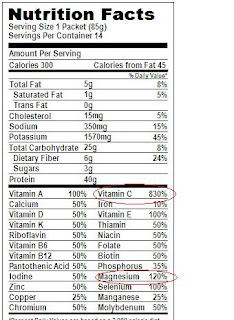- Receipts (taxes)question 16.
Suppose one large square represents 100%. The square is divided into smaller equal-sized pieces.

a.) If there are 1000 pieces, what percent do 17 pieces represent?b.) If there are two large squares each divided into ten smaller pieces, what percent do 13 pieces represent ?c.) If the large square is divided into eight smaller pieces, show how to represent 87 1/2 and 56 1/4.## Wednesday, January 13, 2010

### Gail's Percent blog for January 13, 2010The order of the pictures from top to bottom are #1, #5, #12,and #13 from pages 128-129. Click the picture to make them larger... (:
Thanks and dont forget to comment [:
PEACE OUT [:

## Tuesday, January 12, 2010

### RAQUEL'S SCRIBE FOR PERCENT.

DURING MATH CLASS
So, during math class we corrected the fractions we did for homework. Such as..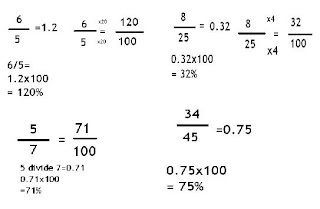We also had to use a 10x10 grid for solving percent problems. such as this:After that, we did a foldable. To do that we had to get 3 pieces of loose leaf and make each one of them evenly spaced out. When you do that you have to fold it backwards and then you will make your foldable which looks like this. :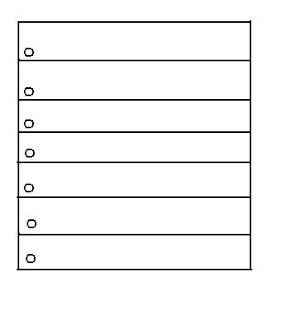(There's to much flaps but it's okay. (: Just imagine there's only 6.)
When your done that, you write what each flap represent. After that, you have to write on the first flap. We had to find the definition of percent and fractional percent. Under that we had to draw out what the percentages ( I think I forgot my foldable at school so, I don't remember the numbers. ) look like on a grid.
The definitions are:

Percent- is a way of expressing a number as a fraction of 100.
Fractional Percent- a percent that includes a portion of a percent.

YES, IT'S LATE WELL.. NOT REALLY BUT, I STILL DID IT. SORRY !! (: ANYWAYS, TELL ME IF I MADE A MISTAKE OR I MISSED SOMETHING, I'LL FIX IT RIGHT AWAY. PC OUT. REMEMBER TO COMMENT.
- Raquel Lorenzo January 12, 2009. (:

## Monday, January 11, 2010

### Jem's Percent

PERCENTS

For todays scribe post its about percent. The task was to be able to solve the given fractions and convert them into percents in 2 different ways so here are my ways :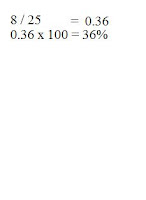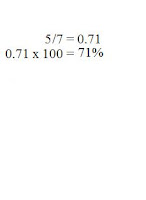## Thursday, January 7, 2010

### Eric.P's scribepost for January 6, 2010

a² + b² = c²
25² + 30² = c²
(25x25)+(30x30) = c²
625 + 900 = c²
1525 = c²
√1525 = √c²
39.05 = c²Thank Christine for the pictures.

### Textbook questions 15 RichardA^2+B^2=C^2
2^2+4^4=C^2
(2x2)+(4x4)=C2
4+16=C2
20=C2
20^(1/2)=C
Therefore the length of the line segment AB is approximately 4 cm.

## Wednesday, January 6, 2010

### Kisha's scribepost for Jan 6

question # 7
What is the height of the wheelchair ramp? Give your answer to the nearest tenth of a centimeter.The height of the ramp is 12.6 cm tall.

c²-b²=
80cm² -79cm²=
(80x80)-(79x79)=
6400cm-6241cm=
159cm=
√159=√a²
12.6cm=a

### Christine's Scribepost for Jan. 6a² + b² = c²
25² + 30² = c²
(25x25)+(30x30) = c²
625 + 900 = c²
1525 = c²
√1525 = √c²
39.1 = c²Shahriar was right, it wasnt as large as the ad said.

### Abigail's Pay It Forward

Part 1
"What is "Pay it Forward"
- To me, Pay it Forward is not just a movie. It's a movie that help use become better citizen, better people, and it als help us understand what the Earth really is.

Part 2
What was your Pay It Forward act of kindness?
- Me, Caitlin, Vicky, Christine, Kim and Razel donated some stuff to the children's hospital. Caitlin, Vicky, Christine, and Kim bought stuff for the goody bags, I bought new stuff toys and Razel donated used toys.

Why did you choose this activity?
- We chose this activity because its the activity that we all agree to do. And we also chose this because we wanted to give the children in the hospital who feel hopeless a hope.

Who did you help?
- We helped the children who are sick in the Children's Hospital.

What did you do?
- We looked for some stuff that just collects dust at home that we dont really use and decided to give them to the sick children. We also bought stuff for them.

When did you do you act of kindness?
- We did it with Mr. McLean . I dont remember whens the exact day we did it but it was a Monday at noon.

Part 3
How did your act of kindness go?
- Our act of kindness went really well and Im not kidding about that. LOL [:

What Happened?
- At 11:35 we left the class were in, science, to get ready because we need to be in room 3 at exactly 11:45. When we get to Mr. McLean's room, we told him were ready to go. After we told him that were ready, he started the car. Then we went in. Mr McLean and Caitlin are in the front and me, Kim, Christine, and Vicky squished ourselves on the back seat LOL [:. At first we cant find where to give the stuff were going to donate, so Mr. McLean went out the car and went inside a building. He asked for direction I think. When he got back, he went inside he car and told us that he now know where the children's hospital is. When we got to the Children's Hospital, Me, Caitlin, Christine, Vicky and Kim went inside and asked a nurse where we could drop our donations. She said that she'll just take it because the other nurse who take the donation is not there right now or maybe she's busy. We gave it to her and she asked us t write a letter for the other nurse and tell them who we are and to write our address so they could write back. We wrote our names, explain what pay it forward is and we wrote our school's address. The nurse said thank you to us and she said that they really appreciate it. We said youre welcome and asked them to do the same. The we went back to Mr. McLean's car, and went back to school. Mr. McLean gave as lollipops on theway back :D . When we reached the school, we went out and have lunch. ( sorry my story telling doesn't make sense)

How did you feel?
- I felt so good, I think the others did too.

How did the person or people react?
- They were so pleased with all our donations. They were shocked of how many we gave.

Did you ask the person or people to "Pay It Forward"?
- Yup, we asked them to pay it forward (:. We wrote it in the letter too. And they said that they will. (:

How did they react to your request?
- They feel.. uhmm.. i dont exactly know how they all reacted because we only told one nurse and we didn't see the other nurse that we wrote a letter to. But the nurse we saw said ok with a smile on her face. (:

Part 4
Why is the idea of "Pay it Forward" important?
- The idea of doing Pay it Forward is important because it will give everyone a change to help even in just a little way. Because if what he/she did think that he/she only did a little, to others it means a LOT.

Has your act of kindness made a difference?
- I think the act of kindness we did made a difference. Im not really sure but that's what I think and hope. (:

### Vicky's Scribe for Question #14

Find the missing side length of each triangle.

A)### Camille's scribe post for January 6, 2010

math text book question 7

7. What is the height of the wheelchair ramp? Give your answer to the nearest tenth of a centimetre.

c2 - b2 = a2
802 - 792 = a2
(80x80) - (79-79) = a2
6400 - 6241 = a2
159 = a2
√159 = √a2
12.6 cm= a

The height of the wheelchair ramp would be 12.6 cm tall

REMEMBER TO LEAVE A COMMENT AND STUDY FOR THE MATH QUIZ !

### Allen's scribepost for January 6, 2010A) a² + b² = c²
5
² + 12² = c²
(5x5) + (12x12) = c
²
25 + 144 = c
²
169 = c
²
√169 = √c²
13 = c

B) c
² - b² = a²
15
² - 9² = a²

(15x15) - (9x9) = a²
225 - 81 = a²
144 = a²
√144 = √a²
12 = a

### Darnell's scribepost for January 5, 201010. a² + b² = c²

27² + 27² = c²
(27x27) + (27x27)= c²
729 + 729 = c²## Tuesday, January 5, 2010

### Scribe,number 9

9.Tina wants to construct a path along the diagonal of her yard. what lenth wil the path be? express the answer to the nearest tenth of a metre.
The lenth will be 24.
Coment!(:

### Michael Rivero's Scribepost Jan. 5, 200912.The Hypotenuse of the triangle cuts the circle in half.What is the diameter of the circle?Express answer to nearest tenth of a centimeter

A²+B²=C²
7²+5²=C²
(7x7)+(5x5)=C²
49+25=C²
Square root of 74=C²
8.6=C²
8.6=Diameter

The diameter of the circle is 8.6 cm

### Kim's Scribepost for 01/05/10In order to find the base of this triangle, add up both high numbers, then take away lowest one, for my answer, I got 12mm.

10+10-8=12

The length of the base of the large triangle is 12mm.

### Razel's Scribepost for January 5, 2010Answer:
First you have to find the side length of the square..( 30.23). So the hypotenuse is 30.23 and the one leg is 17 cm, now I have to find the other side.

c²-a²= b²
30.23² - 17² = b²
(30.23x30.23)-(17x17)= b²
913.85-289=b²
624.85=b²

624.85=b²
√624.85=√b²
24.99cm= b

P= a+b+c
=17+24.99+30.23
= 72.22 cm
The perimeter of the triangle is 72.2

### Caitlin T's Scribepost for January 5, 2010CLICK ON IMAGE TO SEE BETTER!!! and the answer for the second one is actually 7.2**

## Monday, January 4, 2010

### Raquel's Scribepost for Janurary 4 2010

Text Book Questions
Pg.104 Questions 3-4a)12cm-144cm
16cm=256cm
144 cm² + 256 cm² = 400 cm²
Square of 400 cm² = 20 cm

b)16 m = 256 m²

30 m = 900 m²

256 m² + 900 m² = 1156 m²
Square of 1156 m² = 34 ma)7 cm = 49 cm²
6 cm = 36 cm²
49 cm² + 36 cm² = 85 cm²
Square of 85 cm² = 9.2 cm

b)
11 cm = 121 cm²
8 cm = 64 cm²
121 cm² + 64 cm² = 185 cm²
Square of 185 cm² = 13.6 cm

I know it's late but, I still did it. Anyways, COMMENT. (: